# Data Types in java

## 1- Overview of data types

Any programming language has a set of data types. Data types are basic, and quite similar for all languages. All data types are composed from bits, therefore, I dedicate a post to introduce the history of bits and bytes. My advice is that you should read it before continuing reading this post.
Java has two types of data types:
• Primitive Types
• Reference Types
There are 8 primitive data types which are boolean, byte, char, short, int, long, float, double.
Data type Default Value Size
boolean false 1 bit
char '\u0000' 2 byte
byte 0 1 byte
short 0 2 byte
int 0 4 byte
long 0L 8 byte
float 0.0f 4 byte
double 0.0d 8 byte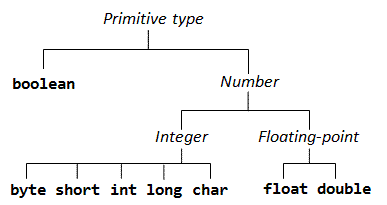• Logic type: boolean.
• Integer types: byte, short, char, int, long.
• Real number type is also called floating point: float, double.
Reference types, which are objects created by the constructor of classes.

## 2- byte

1 byte a byte is composed of 8 consecutive bits in the memory of computer. Each bit  is a binary number of 0 or 1. Java uses " byte" to name a integer type with small scope (Size: 1  byte).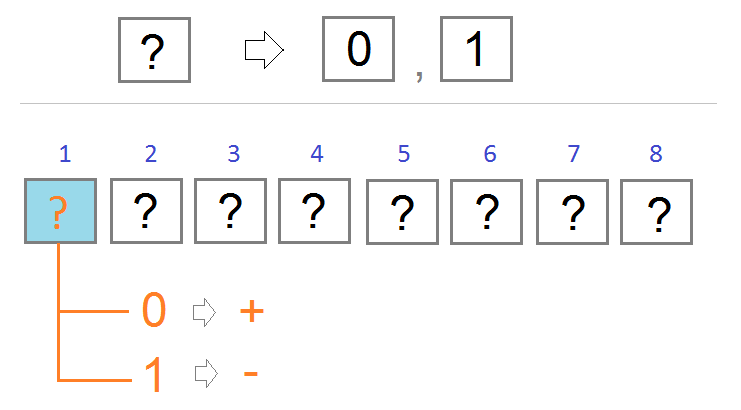The first bit in a row of 8 bits has value of 0 or 1.
• If it is 0, Java considers it as + (Represent a positive number)
• If it is 1 Java considers it as - (Represent a negative number)
For next 7 bits, you can represent a number between 0 and 127. From here you deduce the byte type in Java with the range of [-127, 127].
But wait, it is supposed to be [-128, 127], why?

### Why is the smallest number of byte type in Java -128?

If the rules that the first bit has a value of 0 equivalent to +, the value of 1 equivalent to -, then we have two ways to represent 0 (see the illustration).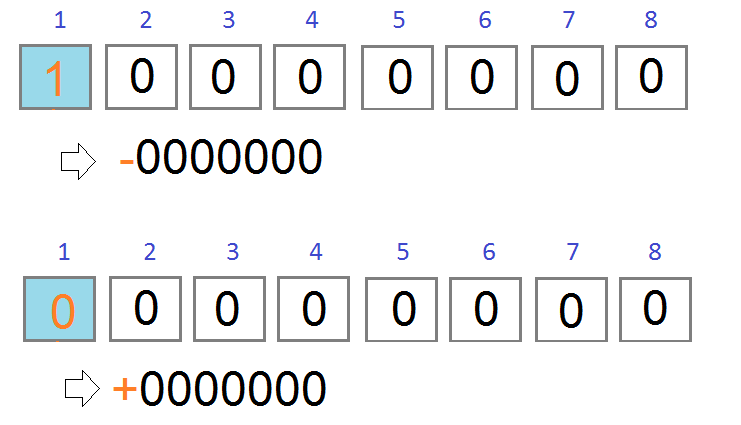Therefore, the binary sequence of  "1 0 0 0 0 0 0" should be considered as the smallest number of byte data type in Java. It represents  -128.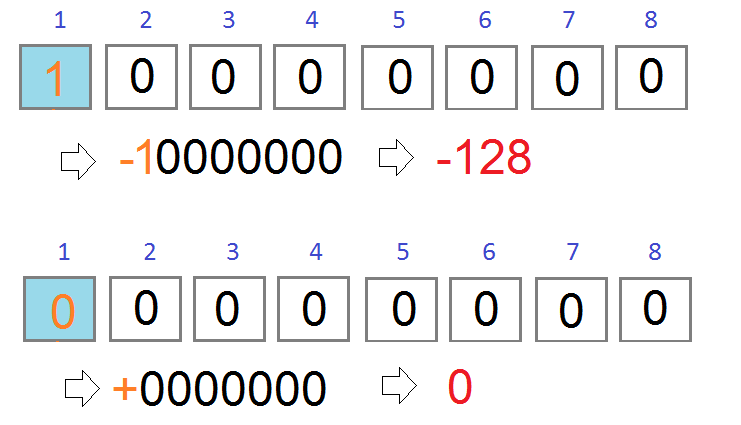## 3- boolean

boolean is the simplest data type. It has size of 1 bit. It has 2 values of   true and  false.
The default value for the  boolean type i s  false.
```// Declare a variable named 'valid', data type is boolean
// The value will be false by default.
boolean valid;

// Declare a variable named 'active', data type is boolean
// Value is true.
boolean active = true;
```

## 4- char

Although " char" is the first four characters of the " Character" term, the char type in Java is used to store non-negative integers with the two- byte size. It is also used to represent for a Unicode character because in nature, each character corresponds to a specific number. (This number is understood as the code of character.)
Because  char is non-negative integer type, size: 2 bytes, its scope is [0, 2*16-1]  ( [0, 65535] ).
When char is understood as a  Unicode character, the smallest character is '\u0000' (Mã 0), and the largest character is '\uffff' (code: 65535).

• TODO

## 5- short

short is data type for the purpose of representing a two-byte integer   (16 bits), including negative integer.
• The smallest value is -32,768 (-2^15) and the largest value is 32,767 (2^15 -1).
• Default value is 0.
** Note: See the explanation of the rule to determine the positive or negative number in the section of  byte data type.

## 6- int

int data type is used to represent an integer with the size of 4 bytes (32 bits).
• The smallest value: -2,147,483,648 (-2^31)
• The largest value:  2,147,483,647 (2^31 -1)
• Default value: 0.
In Java, the int data type is considered as default data type for integers. Therefore, if you write 100, Java will create a four- byte memory area for storage. And if you want Java to create an eight- byte memory area to store 100, you have to write 100L. (long is an eight- byte integer type, introduced in the following section).
```int a = 100;
```

## 7- long

The long data type is used to represent integers with the size of  8 bytes (64 bits).
• The smallest value is -9,223,372,036,854,775,808.(-2^63)
• The largest value is 9,223,372,036,854,775,807. (2^63 -1)
• This type is used when a value pattern wider than int is necessary.
• Default value is 0L.
```// You need to add 'L' immediately after 100
// so that Java creates an 8 byte memory area to store 100
long a = 100L;
```

## 8- float

float data type is used to represent a real number with the size of 4 bytes (32 bits).
• The smallest value: -3.4028235 x 10^38
• The largest value:: 3.4028235 x 10^38
• Default value: 0.0f
Example:
```float value = 2.5f;
```

## 9- double

double data type is used to represent a real number with the size of 8 bytes (64 bits). It is the default type for real numbers.
• The smallest value: -1.7976931348623157 x 10^308
• The largest value:  1.7976931348623157 x 10^308
• Default value: 0.0d
Example:
```double a = 2.5d;

// Since doubling is the default type for real numbers,
// You can write more concisely:
double b = 2.5;
```

## 10- Reference Types

In Java, a data type created by a combination of primitive types is called a reference type. The most commonly used reference type is the String, which is a combination of characters.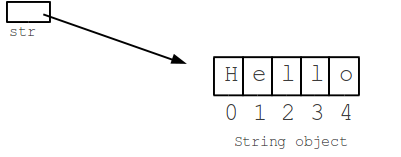Reference types are created based on a class. The class is like a blueprint to define a reference type.
```class Address  {
String cityName;
}

class Student {

String fullName;

int age;

}
```

#### View more Tutorials:

Maybe you are interested

These are online courses outside the o7planning website that we introduced, which may include free or discounted courses.

•Complete Step By Step Java For Testers
•*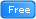* Java Database Connection: JDBC and MySQL
•Java JDBC with Oracle: Build a CRUD Application
•Java Object-Oriented Programming : Build a Quiz Application
•Understanding JDBC with PostgreSQL (A step by step guide)